# ACT Science Practice Test 5

Directions: The ACT Science passage below is followed by several questions. After reading the passage, choose the best answer to each multiple choice question. You may refer to the passage as often as necessary. Calculators may NOT be used test.

Passage V

Suppose a car has an oil leak, such that one drip of oil occurs each second. As the car drives down the road, it will leave a trail of oil behind it which could be used to describe the car’s motion.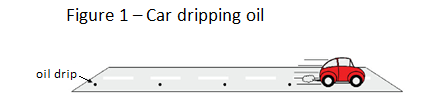Constant Speed
When the car is travelling at constant speed, the oil drips are regularly spaced. The average velocity of the car can be established by taking measurements of the oil drips. The average velocity of the car is equal to the distance traveled divided by the time taken. The car travels at constant speed for the first 10 seconds. Table 1 shows the distance traveled by the car during the first 10 seconds.Acceleration
When an object speeds up, the rate of acceleration can be found by investigating the change in velocity and the time taken to make the change. Table 2 shows the car’s velocity over the next 10 seconds of its motion.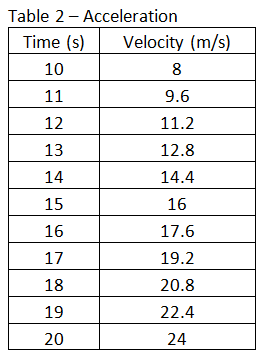Deceleration
When an object slows down, the rate of deceleration can be found by investigating the change in speed and the time taken to make the change. Starting at t = 20 seconds, the driver applies a constant breaking force to bring the car to rest over the next 10 seconds. Figure 2 is a plot of the velocity versus time over the entire duration of the car’s motion from (t=0 to t=30 seconds).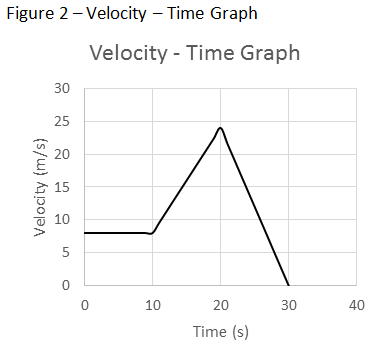Congratulations - you have completed . You scored %%SCORE%% out of %%TOTAL%%. Your performance has been rated as %%RATING%%
 Question 1
What is the velocity of the car at t = 5 seconds?

 A 8 m/s B 16 m/s C 24 m/s D 32 m/s
Question 1 Explanation:
The correct answer is (A). You can read off the car’s velocity at t = 5 seconds from the velocity-time graph in figure 2. At t = 5 seconds, the car’s velocity is 8 m/s.
 Question 2
The car accelerates from t = 10 seconds to t = 20 seconds. What is the car’s acceleration (m/s2) during this period if the rate of acceleration can be found by investigating the change in velocity and the time taken to make the change?

 A 1 B 1.6 C 2.4 D 3.2
Question 2 Explanation:
The correct answer is (B). The acceleration is the change in velocity divided by the time taken to make that change. From Table 2 and Figure 2, we see that the car’s velocity at t = 10 seconds is 8 m/s and the car’s velocity at t = 20 seconds is 24 m/s.Question 3
What is the car’s acceleration (m/s2) during the first 10 seconds of it motion?

 A 0 B 1.6 C 2.4 D 3.2
Question 3 Explanation:
The correct answer is (A). The acceleration is the change in velocity divided by the time taken to make that change. From Figure 2, we see that the car has a constant velocity during the first 10 seconds. In other words, the velocity did not change during the first 10 seconds. Therefore the acceleration is 0 (m/s2).
 Question 4
If instead of applying the brakes at t = 20 seconds, the driver decides to continue driving at a constant speed over the next 10 seconds, which of the following could be the graph of the car’s velocity versus time over the last 10 seconds of its motion?

 AB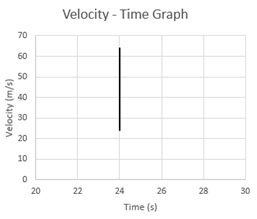C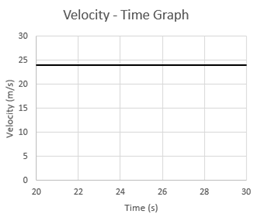DQuestion 4 Explanation:
The correct answer is (C). If the velocity is constant, the graph of velocity versus time will be a straight horizontal line.
 Question 5
If we wish to determine the velocity of the car at t = 20 seconds by investigating the spacing of the oil drops, which of the following describes how we may accomplish this?

 A First determine the distance traveled by the car from t = 0 seconds to t = 20 seconds by measuring the spacing of the oil drops. Then determine the velocity of the car by dividing the distance traveled by the time taken. B First determine the distance traveled by the car from t = 0 seconds to t = 20 seconds by measuring the spacing of the oil drops. Then determine the velocity of the car by multiplying the distance traveled by the time taken. C First determine the distance traveled by the car from t = 10 seconds to t = 20 seconds by measuring the spacing of the oil drops. Then determine the velocity of the car by dividing the distance traveled by the time taken. D First determine the distance traveled by the car from t = 19 seconds to t = 20 seconds by measuring the spacing of the oil drops. Then determine the velocity of the car by dividing the distance traveled by the time taken.
Question 5 Explanation:
The correct answer is (D). Since the car accelerates from t = 10 seconds to t = 20 seconds, we must determine the velocity at t = 20 seconds by investigating the spacing of the oil drops close to t = 20 seconds. If we look at the spacing of the oil drops from t = 19 seconds to t = 20 seconds, we can get an accurate measure of the car’s speed at t = 20 seconds by dividing the distance traveled by the time taken. Answer choice (A) is incorrect because the car does not travel at a constant speed from t = 0 to t = 20 seconds. Answer choice (B) is incorrect for the same reason as in (A) plus the fact that the velocity is not equal to the distance times the time taken as (B) states. Answer choice (C) is incorrect because the car’s speed at t = 10 seconds will be different from its speed at t = 20 seconds since it is accelerating. We can also see this from the velocity time plot, which shows that the speed at t = 10 seconds is 8m/s while the speed at t = 20 seconds is 24 m/s.
 Question 6
Which of the following provides the best description of a plot of the car’s acceleration versus time over the 30 second period studied?

 A An acceleration of 0 m/s2 over the first 10 seconds followed by a positive acceleration over the next 10 seconds, followed by a negative acceleration over the last 10 seconds. B An acceleration of 8 m/s2 over the first 10 seconds followed by a positive acceleration over the next 10 seconds, followed by a negative acceleration over the last 10 seconds. C An acceleration of 0 m/s2 over the first 10 seconds followed by a negative acceleration over the next 10 seconds, followed by a positive acceleration over the last 10 seconds. D An acceleration of 8 m/s2 over the first 10 seconds followed by a positive acceleration over the next 10 seconds, followed by a negative acceleration over the last 10 seconds.
Question 6 Explanation:
The correct answer is (A). The car travels at a constant speed (acceleration = 0 m/s2) over the first 10 seconds. It then accelerates over the next 10 seconds (positive acceleration) before decelerating (negative acceleration) over the last 10 seconds.
Once you are finished, click the button below. Any items you have not completed will be marked incorrect.
There are 6 questions to complete.
 ← List →